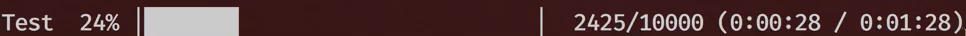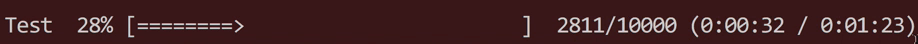Command line progress bar in Java

I have a Java program running in command line mode. I would like to display a progress bar, showing the percentage of job done. The same kind of progress bar you would see using wget under unix. Is this possible?

• I don't know the answer to your specific question, but you could start by checking the libraries mentioned in this question: stackoverflow.com/questions/435740/… – Jonik May 12 '09 at 13:16
• Thanks. The libraries cited there are more about command-line argument parsing, rather than display into the console. But thanks anyways. – g andrieu May 12 '09 at 15:02
• This is on topic. OP asks if possible, not for a library recommendation (at least in current edit) – Neil McGuigan Mar 18 '16 at 22:26
• I try this code stackoverflow.com/questions/1001290/… and running! (Correct running only in terminal, don't use in Eclipse) – Wendel Oct 26 '17 at 13:29

I have implemented this sort of thing before. Its not so much about java, but what characters to send to the console.

The key is the difference between \n and \r. \n goes to the start of a new line. But \r is just carriage return - it goes back to the start of the same line.

So the thing to do is to print your progress bar, for example, by printing the string

"|========        |\r"

On the next tick of the progress bar, overwrite the same line with a longer bar. (because we are using \r, we stay on the same line) For example:

"|=========       |\r"

What you have to remember to do, is when done, if you then just print

"done!\n"

You may still have some garbage from the progress bar on the line. So after you are done with the progress bar, be sure to print enough whitespace to remove it from the line. Such as:

"done             |\n"

Hope that helps.

• Sure helps. Thanks a lot. – g andrieu May 12 '09 at 15:00
• Is this really cross-platform ? It will probably behave ok on Windows and Linux, but what about Macs ? I don't have one, so I can't try it... – Radu Murzea Jun 8 '13 at 15:04
• @RaduMurzea OS X is an *NIX OS, so if it works on Linux, it’ll work on OS X (that’s not true for everything, but that’s true here). – bfontaine Jun 27 '13 at 19:06
• Thanks! However this does not work with ant (tried on both linux and osx; works fine when invoking java directly). Anyone any idea? – user495285 Feb 12 '14 at 20:12
• Note that this does not work in the eclipse built in console (at least for me). But as this is a development-console this should not matter too much. – Qw3ry Feb 7 '17 at 10:47

There is https://github.com/ctongfei/progressbar, License: MIT

Simple console progress bar. Progress bar writing now runs on another thread.Menlo, Fira Mono, Source Code Pro or SF Mono are recommended for optimal visual effects.

For Consolas or Andale Mono fonts, use ProgressBarStyle.ASCII (see below) because the box-drawing glyphs are not aligned properly in these fonts.Maven:

<dependency>
<groupId>me.tongfei</groupId>
<artifactId>progressbar</artifactId>
<version>0.5.5</version>
</dependency>

Usage:

ProgressBar pb = new ProgressBar("Test", 100); // name, initial max
// Use ProgressBar("Test", 100, ProgressBarStyle.ASCII) if you want ASCII output style
pb.start(); // the progress bar starts timing
// Or you could combine these two lines like this:
//   ProgressBar pb = new ProgressBar("Test", 100).start();
some loop {
...
pb.step(); // step by 1
pb.stepBy(n); // step by n
...
pb.stepTo(n); // step directly to n
...
pb.maxHint(n);
// reset the max of this progress bar as n. This may be useful when the program
// gets new information about the current progress.
// Can set n to be less than zero: this means that this progress bar would become
// indefinite: the max would be unknown.
...
pb.setExtraMessage("Reading..."); // Set extra message to display at the end of the bar
}
pb.stop() // stops the progress bar
• this looks neat, and it will allow me to replace a couple hundred string-buffer lines I've got on my CLI. But what if I've got an application that runs for minutes between "ticks" (minutes between step() calls)? Does your library run asynchronously, thus allowing the clock to update? What if my library runs for days? Does it use the /r strategy? Will that result in multi-hundred-megabyte output? – Groostav May 9 '18 at 17:31

I found the following code to work correctly. It writes bytes to the output buffer. Perhaps that methods using a writer like the System.out.println() method replaces the occurrences of \r to \n to match the target's native line ending(if not configured properly).

public class Main{
public static void main(String[] arg) throws Exception {
String anim= "|/-\\";
for (int x =0 ; x < 100 ; x++) {
String data = "\r" + anim.charAt(x % anim.length()) + " " + x;
System.out.write(data.getBytes());
}
}
}

I have made a percentage progress bare to check the remain download file.

I call the method periodically in my file download to check the total file-size and remaining and present that in %.

It can be used for other task purpose as well.

Test and output example

progressPercentage(0, 1000);
[----------] 0%

progressPercentage(10, 100);
[*---------] 10%

progressPercentage(500000, 1000000);
[*****-----] 50%

progressPercentage(90, 100);
[*********-] 90%

progressPercentage(1000, 1000);
[**********] 100%

Test with for loop

for (int i = 0; i <= 200; i = i + 20) {
progressPercentage(i, 200);
try {
} catch (Exception e) {
}
}

The method can be easily modified:

public static void progressPercentage(int remain, int total) {
if (remain > total) {
throw new IllegalArgumentException();
}
int maxBareSize = 10; // 10unit for 100%
int remainProcent = ((100 * remain) / total) / maxBareSize;
char defaultChar = '-';
String icon = "*";
String bare = new String(new char[maxBareSize]).replace('\0', defaultChar) + "]";
StringBuilder bareDone = new StringBuilder();
bareDone.append("[");
for (int i = 0; i < remainProcent; i++) {
bareDone.append(icon);
}
String bareRemain = bare.substring(remainProcent, bare.length());
System.out.print("\r" + bareDone + bareRemain + " " + remainProcent * 10 + "%");
if (remain == total) {
System.out.print("\n");
}
}
• Thank you. I've updated your code – see below. – Dennis Mar 23 at 13:36

C# Example but I'm assuming this is the same for System.out.print in Java. Feel free to correct me if I'm wrong.

Basically, you want to write out the \r escape character to the start of your message which will cause the cursor to return to the start of the line (Line Feed) without moving to the next line.

static string DisplayBar(int i)
{
StringBuilder sb = new StringBuilder();

int x = i / 2;
sb.Append("|");
for (int k = 0; k < 50; k++)
sb.AppendFormat("{0}", ((x <= k) ? " " : "="));
sb.Append("|");

return sb.ToString();
}

static void Main(string[] args)
{
for (int i = 0; i <= 100; i++)
{
Console.Write("\r{0} {1}% Done", DisplayBar(i), i);
}

}
• Nice example, will sure be helpful. Thanks. – g andrieu May 12 '09 at 15:01
• Beautiful! Thank you. Works like a charmer. Easy to read. Lovely – kholofelo Maloma Jul 7 '16 at 9:55

Here is a modified version of the above:

private static synchronized void loading(String msg) throws IOException, InterruptedException {
System.out.println(msg);
@Override
public void run() {
try {
System.out.write("\r|".getBytes());
System.out.write("-".getBytes());
}
System.out.write("| Done \r\n".getBytes());
} catch (IOException e) {
e.printStackTrace();
} catch (InterruptedException e) {
e.printStackTrace();
}
}
};
th.start();
}

... and in main:

• This is a nice example of Multithreading ! Very helpful. – LMD May 20 '17 at 20:29

This would be possible with a Java Curses library. This is what I have found. I haven't used it myself and I don't know if it is cross-platform.

• Curses may be a bit of overhead for the easy usage I need, but that sure is a track. Thanks. – g andrieu May 12 '09 at 15:03

I use a "bouncing" progress bar when I need to delay a tool to prevent a race condition.

private void delay(long milliseconds) {
String bar = "[--------------------]";
String icon = "%";

long startTime = new Date().getTime();
boolean bouncePositive = true;
int barPosition = 0;

while((new Date().getTime() - startTime) < milliseconds) {
if(barPosition < bar.length() && barPosition > 0) {
String b1 = bar.substring(0, barPosition);
String b2 = bar.substring(barPosition);
System.out.print("\r Delaying: " + b1 + icon + b2);
if(bouncePositive) barPosition++;
else barPosition--;
} if(barPosition == bar.length()) {
barPosition--;
bouncePositive = false;
} if(barPosition == 0) {
barPosition++;
bouncePositive = true;
}

try { Thread.sleep(100); }
catch (Exception e) {}
}
System.out.print("\n");
}

I have recently faced the same problem, you can check my code: I have set it for one # on 5%, which you can modify later.

public static void main (String[] args) throws java.lang.Exception
{
int i = 0;
while(i < 21) {
System.out.print("[");
for (int j=0;j<i;j++) {
System.out.print("#");
}

for (int j=0;j<20-i;j++) {
System.out.print(" ");
}

System.out.print("] "+  i*5 + "%");
if(i<20) {
System.out.print("\r");
}
i++;
}
System.out.println();
}

A bit refactored and updated @maytham-ɯɐɥʇʎɐɯ 's method. Now it's supporting an arbitrary size of the progress bar:

public static void progressPercentage(int done, int total) {
int size = 5;
String iconLeftBoundary = "[";
String iconDone = "=";
String iconRemain = ".";
String iconRightBoundary = "]";

if (done > total) {
throw new IllegalArgumentException();
}
int donePercents = (100 * done) / total;
int doneLength = size * donePercents / 100;

StringBuilder bar = new StringBuilder(iconLeftBoundary);
for (int i = 0; i < size; i++) {
if (i < doneLength) {
bar.append(iconDone);
} else {
bar.append(iconRemain);
}
}
bar.append(iconRightBoundary);

System.out.print("\r" + bar + " " + donePercents + "%");

if (done == total) {
System.out.print("\n");
}
}
public static void main(String[] argv) throws Exception{

System.out.write("\r".getBytes());
int percentage =10;
while(percentage <= 100) {
String temp =generateStars(percentage);
System.out.write(temp.getBytes());
System.out.print("\b\b\b");
percentage = percentage+10;
}
}

public static String generateStars(int percentage)
{
int startsNum = percentage / 4;
StringBuilder builder = new StringBuilder();
while(startsNum >= 0)
{
builder.append("*");
startsNum--;
}
builder.append(percentage+"%");
return builder.toString();
}
public class ProgressBar
{
private int max;

public ProgressBar(int max0) {
max = max0;
update(0);
}

public void update(int perc) {
String toPrint = "|";
for(int i = 0; i < max; i++) {
if(i <= (perc + 1))
toPrint += "=";
else
toPrint += " ";
}

if(perc >= max)
Console.print("\r");
else
Console.print(toPrint + "|\r");
}
}
public class Main {

public static void main(String[] args) throws Exception {

int count =1;
for(int j=1;j<150;j++){

System.out.print("\r");
if(count==1){
System.out.print("/");
count++;
}
else if(count==2){
System.out.print("|");
count++;
}
else if(count==3){
System.out.print("-");
count++;
}
else if(count==4){
System.out.print("\\");
count++;
}
else if(count==5){
System.out.print("|");
count++;
}
else
count = 1;